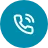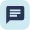# How to Calculate Percentage in Excel

#### Subscribe

One of the essential features of Microsoft Excel is the ability to perform calculations and analyze data using formulas and functions. Percentages are often used in Excel to represent data in the form of ratios or proportions. Whether you’re working on a budget, analyzing sales data, or calculating employee performance, knowing how to use percentages in Excel is an essential skill that can help you save time and improve accuracy.

In this blog, we will explore the basics of percentages, how to calculate percentages in Excel sheet, how to calculate discount in Excel and how to calculate percentage increase in Excel amongst others. Keep reading to find out.## Step-By-Step Procedure of Calculating Percentage in MS Excel

Calculating percentages in Microsoft Excel is a relatively simple process that involves a few basic steps. Here’s a step-by-step guide on how to calculate percentages in Excel:

The first step is to enter the data you want to calculate the percentage for into Excel. For example, if you’re calculating a percentage increase in sales, you would enter the sales figures for the two periods you want to compare.

Step 2: Select the cell where you want to display the percentage

Select the cell where you want to display the percentage result. This cell will be where the formula is entered.

Step 3: Enter the formula

Enter the formula to calculate the percentage in the selected cell. The formula is as follows:

= (new value – old value) / old value * 100

In this formula, “new value” represents the updated or increased value you’re comparing to the “old value” of the same data set. The result of this formula is the percentage increase or decrease between the two values.

Step 4: Format the cell

Format the cell where the percentage result appears as a percentage. This can be done by selecting the cell and using the “Format Cells” option from the right-click menu. Under the “Number” tab, select “Percentage” from the “Category” list and set the number of decimal places you want to display.

Step 5: Check the result

Check the result to ensure that the calculation is correct. If the result is incorrect, double-check the formula to ensure that it’s accurate and that the data is entered correctly.

That’s it! Wasn’t that easy? Following these simple steps will enable you to calculate percentages in Excel quickly and accurately. Now that you know what is are basic , let’s talk about the Fundamentals of Calculating Percentage.

## Fundamentals of Calculating Percentages in MS Excel

The fundamentals to calculate percentages in Excel are based on the use of simple arithmetic operations and the application of the correct formatting functions. Here are some of the basic fundamentals to keep in mind when calculating percentages in Excel:

• Understanding the formula:
The percentage formula in Excel is simple: percentage = (part/whole) * 100. This formula can be used to calculate percentages in many different scenarios.
• Formatting cells:
Formatting cells is an essential part of calculating percentages in Excel. This is because Excel uses a default format that does not display percentages. To change this, you must format the cell as a percentage. This can be done by selecting the cell or range of cells, right-clicking, and choosing “Format Cells”. Under the “Number” tab, select “Percentage” and choose the number of decimal places you want to display.
• Using absolute cell references:
When using a formula that involves dividing a cell value by another, it is essential to use absolute cell references. This is because relative cell references change as you copy the formula to other cells. By using absolute cell references, the formula will always reference the same cells.
• Applying conditional formatting:
Conditional formatting is another essential tool for calculating percentages in Excel. This feature allows you to highlight cells based on their values or conditions. For example, you can use conditional formatting to highlight cells that exceed a certain percentage or to show which cells contain negative values.

By keeping these fundamentals in mind, you can calculate percentages in Microsoft Excel quickly and accurately, helping you make informed decisions based on your data. Let’s look at the percentage formula used in Excel.## What is the Excel percentage formula?

The Excel percentage formula calculates a percentage from two given values: the part (or the new value) and the whole (or the old value). The formula to calculate a percentage in Excel is:

Percentage = (Part / Whole) * 100

The part represents the new value or the value you want to calculate the percentage for, while the whole represents the old value or the original amount. The result of the formula will be a percentage, expressed with the “%” symbol.

For example, if you want to calculate the percentage increase of sales from the first quarter to the second quarter, and your sales figures were 100 in Q1 and 120 in Q2, you can use the formula as follows:

= ((120 – 100) / 100) * 100

This will give you a result of 20, which is the percentage increase in sales from Q1 to Q2. You can then format the cell as a percentage to display the result as “20%”. Lastly, let’s look at the different types of percentages calculating on Ms Excel.

There are several different types of calculating percentages that can be used depending on the specific situation. Here are some of the most common methods for calculating percentages:

• Percentage increase/decrease:
This method calculates the percentage change between two values. To calculate the percentage increase, subtract the original value from the new value, divide by the original value, and multiply by 100. To calculate the percentage decrease, follow the same steps, but subtract the new value from the original value.
• Percentage of the total:
This method calculates the percentage that a particular value represents of the total value. To calculate the percentage of a total, divide the part by the whole and multiply by 100.
• Percentage difference:
This method calculates the percentage difference between two values, where one value is greater than the other. To calculate the percentage difference, divide the absolute difference between the two values by the average of the two values, and multiply by 100.
• Markup and margin percentages:
These methods are used in business and finance to calculate the selling price of a product or service. Markup percentage is the amount added to the cost price to get the selling price, expressed as a percentage of the cost price. Margin percentage is the amount of profit made on a product or service, expressed as a percentage of the selling price.
• Weighted percentage:
This method is used to calculate the percentage contribution of each item in a group, where each item has a different weight or importance. To calculate the weighted percentage, multiply each item by its weight, add the results, and divide by the total weight.

These are just a few of the different methods used for calculating percentages, and the specific method used will depend on the situation and the data available.

In conclusion, working with percentages in Microsoft Excel is an important aspect of data analysis and financial calculations. With the help of formulas and functions, it is easy to calculate various types of percentages in Excel, including percentage increase/decrease, percentage of a total, percentage difference, markup and margin percentages, and weighted percentages. Understanding the fundamental principles of calculating percentages in Excel, such as using absolute cell references, applying conditional formatting, and formatting cells, is essential for accurate and efficient calculations. By mastering these skills, you can unlock the full potential of Excel for your data analysis needs.

Share:

#### Accelerate Your Career with Expert Guidance and Guaranteed Job*!Request a call backLive chat with us